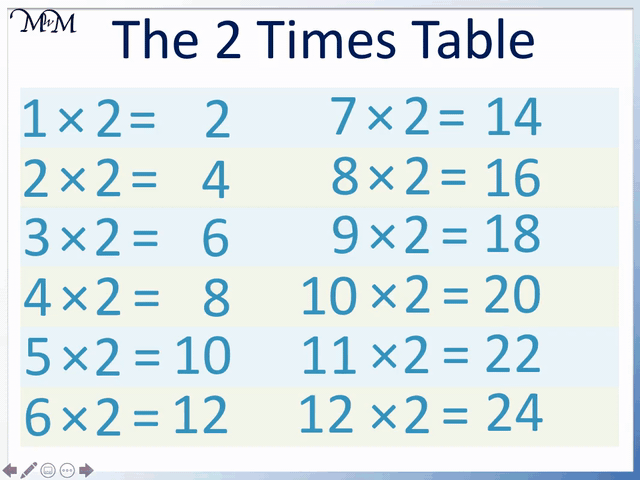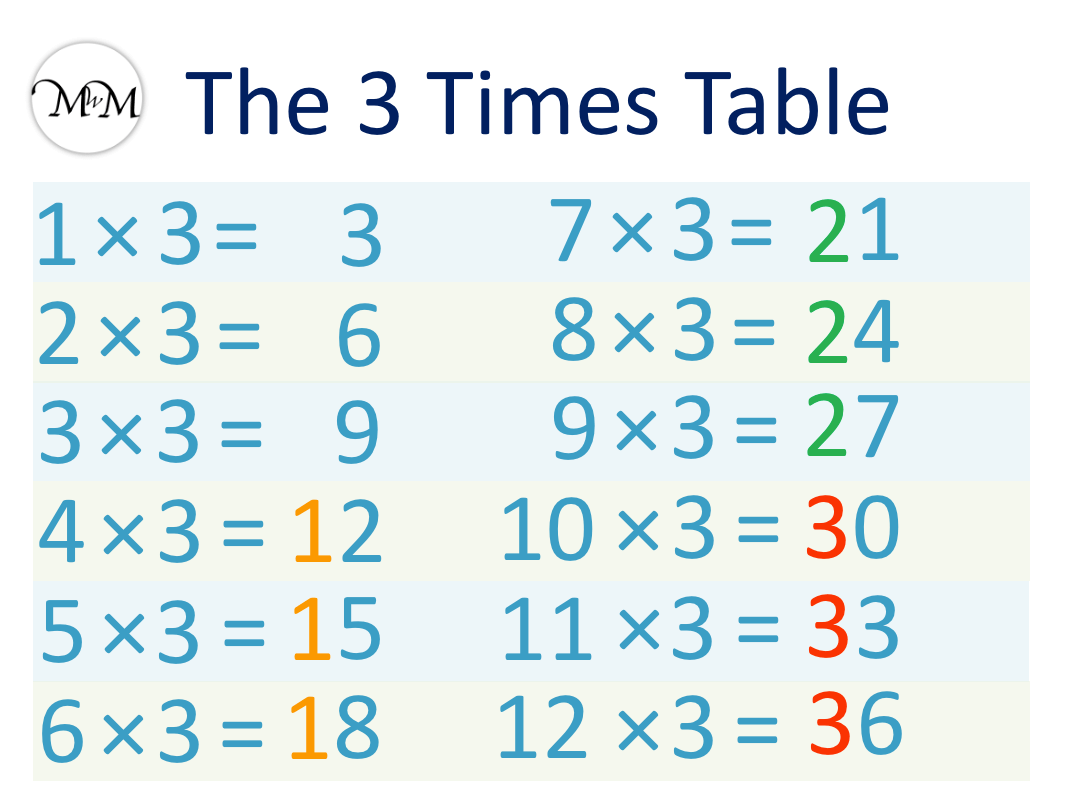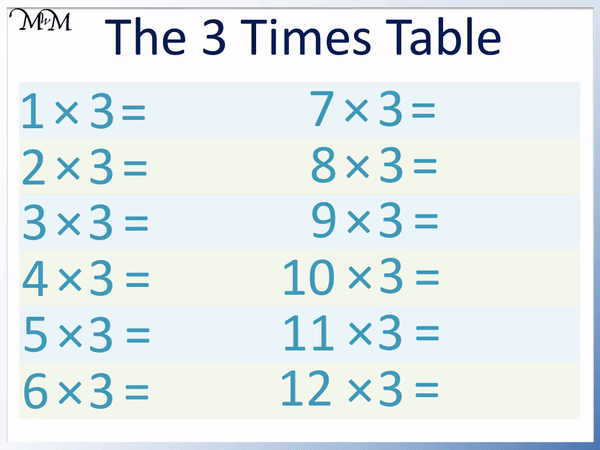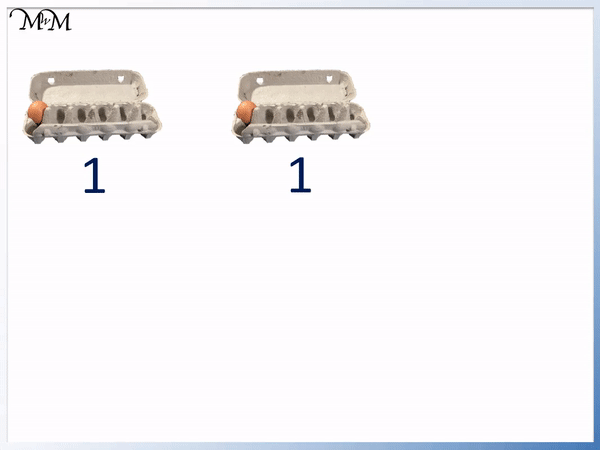# The 3 Times Table

The 3 Times TableThe 3 times table is:

• 1 × 3 = 3
• 2 × 3 = 6.
• 3 × 3 = 9
• 4 × 3 = 12
• 5 × 3 = 15
• 6 × 3 = 18
• 7 × 3 = 21
• 8 × 3 = 24
• 9 × 3 = 27
• 10 × 3 = 30
• 11 × 3 = 33
• 12 × 3 = 36• To learn the three times table, it is helpful to already know the two times table.
• We simply add the number that we are multiplying by 3 onto the answer to the 2 times table.
• For example, 5 × 3 is just another 5 more than 5 × 2.
• If we know that 5 × 2 = 10, we can add 5 to this answer to see that 5 × 3 = 15.
• We can add the digits of the answers to the 3 times table and they will always repeat the pattern of 3, 6, and 9.

To multiply a number by 3, multiply it by 2 and then add the number on to the answer.• To multiply a number by 3, multiply it by 2 and then add the number on to the answer.
• 5 × 2 = 10.
• 10 + 5 = 15 and so, 5 × 3 = 15#### 3 Times Table Flashcards

Click on the 3 times table flashcards below to memorise the 3 times table.

×
×

=

# The 3 Times Table

## What is the 3 Times Table?

The 3 times table starts at three and counts up in threes like so:

• 1 × 3 = 3
• 2 × 3 = 6.
• 3 × 3 = 9
• 4 × 3 = 12
• 5 × 3 = 15
• 6 × 3 = 18
• 7 × 3 = 21
• 8 × 3 = 24
• 9 × 3 = 27
• 10 × 3 = 30
• 11 × 3 = 33
• 12 × 3 = 36

To get from one answer in the 3 times table to the next, simply add three.

The full three times table list is shown below.It is best to learn the three times table after the two times table. Knowing the two times table very well makes it much easier to learn the three times table.

## How to Learn the 3 Times Table

The easiest way to learn the 3 times table is to remember that adding the digits of the numbers gives us the pattern of 3, 6 and then 9. Every time the pattern is repeated, we increase the tens digit by 1 and decrease the ones column by 1.

For example, we have 3, 6 and 9.

Next comes 12, 15 and 18, whose digits add to 3, 6 and 9.

Next comes 21, 24, and 27, whose digits add to 3, 6 and 9.

Finally we have 30, 33 and 36, whose digits add to 3, 6 and 9.We can see that we have groups of three numbers in each ten.

3, 6 and 9 have zero tens.

12, 15 and 18 have one ten.

21, 24 and 27 have two tens.

30, 33 and 36 have three tens.We can see that we increase the tens digit by one each time we have the pattern of 3, 6 and 9.

The digit in the units column decreases by one each time the tens digit increases by one.For example, if we know that we start with 3, 6 and 9, then we next have 12, 15 and 18.

12, 15 and 18 end in 2, 5 and 8 which is one less than 3, 6 and 9.

Next is 21, 24 and 27, which end in 1, 4 and 7. These are one less than 2, 5 and 8 in the previous group.

Finally, 30, 31 and 32 end in 0, 1 and 2, which are one less than 1, 4 and 7 in the previous group.

Provided that we remember the 3, 6, 9 pattern, we can learn the 3 times table quite quickly. The numbers come in groups of three with their digits adding to 3, 6 and 9. Every time that we repeat this pattern, the tens column increases by 1 and the ones column decreases by 1.

## Teaching the 3 Times Table

The above trick is the easiest method for learning the three times table, however when first introducing it, it is helpful to remember that it is finding three lots of a number.

For example, 3 × 5 means 5 + 5 + 5. We add 5 three times.

If we already know the 2 times table, then we already have two lots of the number and we only need to add one more lot.

We can teach the three times table using the two times table. If we know our two times table well, we can simply add another lot on to the answer.

For example, here is 3 × 2.

We already know that 2 × 2 = 4 from our two times table.We can add another 2 onto 4 to get 3 × 2 = 6.

2 × 2 means 2 + 2 and 3 × 2 means 2 + 2 + 2. We only have to add on another 2.

Here is another example of 5 × 3.

From the two times table, 5 × 2 = 10.We add another lot of 5 to our answer. 10 + 5 = 15 and so, 5 × 3 = 15.

Here is the full three times table shown below, where each number is added on to the two times table.whilst not the quickest method, this strategy can be useful to teach to help reinforce that if we know one times table, it can be used to find another times table that is close by.

The two times table is one of the easier ones to learn as it is simply doubling each number by adding it to itself. This means that it is one of the first times tables to be learnt and most children will learn this first.

If they remember the two times table, they can work out the threes by adding on the number again.

Overall, it is best to know the times tables by heart and not have to work them out. This is because there are many aspects of Mathematics that require times tables and knowing them inside out allows us to spend less times focusing on the times table and more time on the new methods we are learning.

The best way to remember the three times table is the pattern of 3, 6, 9 shown above.The numbers in the three times table are always odd.Now try our lesson on Multiplying by 0 and 1 where we learn how to multiply by 0 and 1.error: Content is protected !!